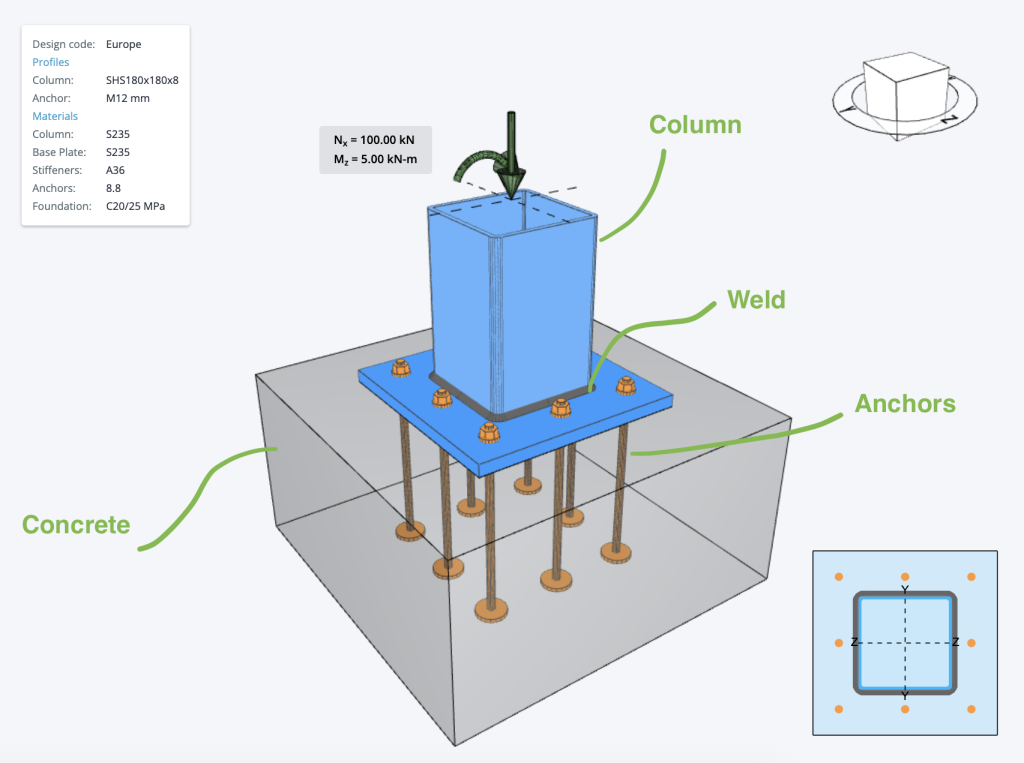SkyCiv文档

1. SkyCiv连接设计
2. 底板设计
3. AISC 钢底板设计示例 美国规范

# AISC 底板设计示例 美国规范

• 下面是一些在底板设计中常用的美国底板计算示例 – 下面是一些在底板设计中常用的美国底板计算示例 318
• 下面是一些在底板设计中常用的美国底板计算示例 – 下面是一些在底板设计中常用的美国底板计算示例, 下面是一些在底板设计中常用的美国底板计算示例 360
• 下面是一些在底板设计中常用的美国底板计算示例 – 下面是一些在底板设计中常用的美国底板计算示例, 下面是一些在底板设计中常用的美国底板计算示例
• 下面是一些在底板设计中常用的美国底板计算示例 (柱) 支票 – 下面是一些在底板设计中常用的美国底板计算示例## 荷载组合:

1. $$1.4D) 2. \(1.2d + 1.6大号 + 0.5(L_{[R} \文本{ 要么 } S 文本{ 要么 } [R)$$
3. $$1.2d + 1.6(左文本{ 要么 } S 文本{ 要么 } [R) + (L 文本{ 要么 } 0.5w ^)$$
4. $$1.2d + 1.0w ^ + 大号 + 0.5(左文本{ 要么 } S 文本{ 要么 } [R)$$
5. $$1.2d + 1.0Ë + 大号 + 0.2S) 6. \(0.9d + 1.0W) 7. \(0.9d + 1.0E) 哪里 : \(D) = 静载 \(L) = 活荷载 \(L_{[R}$$ = 屋顶活荷载
$$S) = 雪荷载 \(R) = 雨量 \(E) = 地震 \(W) = 风荷载 ### 包括详细的分步计算: ## ACI 混凝土轴承检查: 下面是一些在底板设计中常用的美国底板计算示例 包括详细的分步计算 (压缩) 包括详细的分步计算 360-16 情商. J8-2. \( F_{b} = phi _{轴承} \次 0.85 \次 f’_{C} \次 sqrt{ \压裂{ 一个_{2} }{ 一个_{1} } } \leq F_{b, 限制} = 1.70 \倍f_{C} \次A_{1}$$

$$F'_{C}$$ 包括详细的分步计算
$$一个_{1}$$ – 与混凝土表面接触的底板区域
$$一个_{2}$$ – 混凝土支撑面
$$\phi_{轴承}$$ – 混凝土的阻力系数 ( 默认值= 0.65 )

## AISC 焊接设计检查:

$$(一世) R_{ñ} = R_{空值} + R_{净重}$$

$$(ii) R_{ñ} = 0.85R_{空值} + 1.5R_{净重}$$

$$R_{空值}$$ = 纵向加载角焊缝的总标称强度.
$$R_{净重}$$ = 横向加载角焊缝的总标称强度.

## ACI 锚设计检查:

• 锚杆的拉剪钢强度, $$\φN_{至}$$ 和 $$\非V_{至}$$.
• 混凝土抗拉强度和抗剪强度, $$\φN_{背景}$$ 和 $$\非V_{背景}$$.
• 混凝土抗拔强度, $$\φN_{p}$$.
• 混凝土侧面爆破强度, $$\φN_{某人}$$.
• 锚杆在剪切中的混凝土顶出强度, $$\非V_{cp}$$.

#### 锚杆的拉剪钢强度

$$\φ_{紧张, 无} N_{至} = phi _{紧张, 无} 一个_{我知道,ñ}F_{乌塔} \右箭头$$ 方程 17.6.1.2

$$\φ_{剪力, 无} V_{至} = phi _{剪力, 无} 0.6一个_{我知道,V}F_{乌塔} \右箭头$$ 等式 17.7.1.2b

• $$\φ_{紧张, 无}$$ – 受拉锚的强度折减系数 ( 默认值 = 0.75 )
• $$\φ_{剪力, 无}$$ – 剪力锚的强度折减系数 ( 默认值 = 0.65 )
• $$一个_{我知道,ñ}$$ – 是受拉锚的有效横截面积.
• $$一个_{我知道,V}$$ – 是锚杆在剪切时的有效横截面积.
• $$F_{乌塔}$$ 包括详细的分步计算 $$1.9F_{是的}$$ 和 125 KSI (861.845 兆帕)

#### 混凝土抗裂强度

$$\φN_{背景} = phi frac{ 一个_{数控} }{ 一个_{记住} } \psi_{欧共体,ñ} \psi_{编辑,ñ} \psi_{C,ñ} \psi_{cp,ñ} N_{b} \右箭头$$ 等式 17.6.2.ab

$$\φ$$ – 受拉锚的强度折减系数 ( 默认值 = 0.75 ).
$$一个_{数控}$$ – 单个或一组锚栓的预计混凝土破坏.
$$一个_{记住}$$- 单个锚杆的工程混凝土破坏区域, 如果不受边缘距离或间距的限制，则用于计算拉伸强度.

$$\psi_{欧共体,ñ}$$ – 张力中的突围偏心率因素.

$$\磅/平方英寸{欧共体,ñ} = frac{1.00}{ 1 + \压裂{e^{‘}_{ñ}}{1.5 H_{ef}} } \列克 1.00 \右箭头$$ 方程 17.6.2.3.1

$$\psi_{编辑,ñ}$$ – 张力中的突围效应因子.

(一个) $$\文本{如果 } C_{一个,分} \geq 1.5h_{ef} \文本{ 然后 } \磅/平方英寸{编辑,ñ} = 1.00$$ 等式 17.6.2.4.1a

(b) $$\文本{如果 } C_{一个,分} < 1.5H_{ef} \文本{ 然后 } \磅/平方英寸{编辑,ñ} = 0.70 + 0.3\压裂{C_{一个,分}}{1.5H_{ef}}$$ 等式 17.6.2.4.1b

$$\psi_{C,ñ}$$ – 张力中的断裂开裂因素.

$$\磅/平方英寸{C,ñ} = 1.25$$ 用于预制锚栓

$$\psi_{cp,ñ}$$ – 张力中的突破分裂因子.

(一个) $$\文本{如果 } C_{一个,分} \geq C_{交流电} \文本{ 然后 } \磅/平方英寸{cp,ñ} = 1.00$$ 等式 17.6.2.4.1a

(b) $$\文本{如果 } C_{一个,分} < C_{交流电} \文本{ 然后 } \磅/平方英寸{cp,ñ} = frac{ C_{一个,分} }{ C_{交流电}} \geq 压裂{ 1.5H_{ef} }{ C_{交流电} }$$ 等式 17.6.2.4.1b

$$N_{b}$$ – 开裂混凝土中单个锚杆受拉时的基本混凝土断裂强度.

#### 混凝土抗拔强度

ACI 中定义了锚杆的系数混凝土抗拔强度 318-19 – 17.6.3 作为

ϕNpn = φΨC,P ñp

$$\φ$$ – 受拉锚的强度折减系数 ( 默认值 = 0.70 ).
$$\磅/平方英寸{C, P}$$ – 混凝土条件修正系数

$$\磅/平方英寸{C, P}$$ = 1.0

$$\磅/平方英寸{C, P}$$ = 1.4

$$N_{p}$$ – 锚拉拔强度

$$N_{p} = 8A_{brg}f^{‘}_{C}$$ 等式 17.6.3.2.2a

$$N_{p} = 0.9f^{‘}_{C}e_{H}d_{一个} \右箭头$$ 等式 17.6.2.2.b

$$f^{‘}_{C}$$ – 混凝土的规定抗压强度.
$$一个_{brg}$$ – 螺柱头部净承载面积, 地脚螺栓或带头变形棒.
$$e_{H}$$ – 从 J 型螺栓或 L 型螺栓的轴的内表面到 J 型螺栓的外尖端的距离- 或 L 型螺栓.
$$d_{一个}$$ – 锚栓外径或带头螺柱轴径, 带头螺栓, 或钩形螺栓.

#### 混凝土侧面爆破强度

ACI 中定义了锚杆的系数混凝土侧面井喷强度 318-19 – 17.6.4 作为

$$\φN_{某人} = 160C_{a1}\sqrt{一个_{brg}}\拉姆达_{一个} \sqrt{f^{‘}_{C} } \右箭头$$ 方程 17.6.4.1

$$f^{‘}_{C}$$ – 混凝土的规定抗压强度.
$$一个_{brg}$$ – 螺柱头部净承载面积, 地脚螺栓或带头变形棒.
$$\拉姆达_{一个}$$ – 反映在某些混凝土锚固应用中轻质混凝土力学性能降低的修正系数.